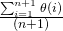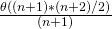# Analysis of Algorithms | Set 2 (Worst, Average and Best Cases)

• Difficulty Level : Easy
• Last Updated : 16 Sep, 2021

In the previous post, we discussed how Asymptotic analysis overcomes the problems of the naive way of analyzing algorithms. In this post, we will take an example of Linear Search and analyze it using Asymptotic analysis.
We can have three cases to analyze an algorithm:
1) The Worst Case
2) Average Case
3) Best Case
Let us consider the following implementation of Linear Search.

Attention reader! Don’t stop learning now. Get hold of all the important DSA concepts with the DSA Self Paced Course at a student-friendly price and become industry ready.  To complete your preparation from learning a language to DS Algo and many more,  please refer Complete Interview Preparation Course.

In case you wish to attend live classes with experts, please refer DSA Live Classes for Working Professionals and Competitive Programming Live for Students.

## C++

 `// C++ implementation of the approach``#include ``using` `namespace` `std;` `// Linearly search x in arr[].``// If x is present then return the index,``// otherwise return -1``int` `search(``int` `arr[], ``int` `n, ``int` `x)``{``    ``int` `i;``    ``for` `(i = 0; i < n; i++) {``        ``if` `(arr[i] == x)``            ``return` `i;``    ``}``    ``return` `-1;``}` `// Driver Code``int` `main()``{``    ``int` `arr[] = { 1, 10, 30, 15 };``    ``int` `x = 30;``    ``int` `n = ``sizeof``(arr) / ``sizeof``(arr);``    ``cout << x << ``" is present at index "``         ``<< search(arr, n, x);` `    ``getchar``();``    ``return` `0;``}` `// This code is contributed``// by Akanksha Rai`

## C

 `// C implementation of the approach``#include ` `// Linearly search x in arr[].``// If x is present then return the index,``// otherwise return -1``int` `search(``int` `arr[], ``int` `n, ``int` `x)``{``    ``int` `i;``    ``for` `(i = 0; i < n; i++) {``        ``if` `(arr[i] == x)``            ``return` `i;``    ``}``    ``return` `-1;``}` `/* Driver program to test above functions*/``int` `main()``{``    ``int` `arr[] = { 1, 10, 30, 15 };``    ``int` `x = 30;``    ``int` `n = ``sizeof``(arr) / ``sizeof``(arr);``    ``printf``(``"%d is present at index %d"``, x,``           ``search(arr, n, x));` `    ``getchar``();``    ``return` `0;``}`

## Java

 `// Java implementation of the approach` `public` `class` `GFG {` `    ``// Linearly search x in arr[].  If x is present then``    ``// return the index, otherwise return -1``    ``static` `int` `search(``int` `arr[], ``int` `n, ``int` `x)``    ``{``        ``int` `i;``        ``for` `(i = ``0``; i < n; i++) {``            ``if` `(arr[i] == x) {``                ``return` `i;``            ``}``        ``}``        ``return` `-``1``;``    ``}` `    ``/* Driver program to test above functions*/``    ``public` `static` `void` `main(String[] args)``    ``{``        ``int` `arr[] = { ``1``, ``10``, ``30``, ``15` `};``        ``int` `x = ``30``;``        ``int` `n = arr.length;``        ``System.out.printf(``"%d is present at index %d"``, x,``                          ``search(arr, n, x));``    ``}``}` `/*This code is contributed by PrinciRaj1992*/`

## Python3

 `# Python 3 implementation of the approach` `# Linearly search x in arr[]. If x is present``# then return the index, otherwise return -1`  `def` `search(arr, x):``    ``for` `index, value ``in` `enumerate``(arr):``        ``if` `value ``=``=` `x:``            ``return` `index``    ``return` `-``1`  `# Driver Code``arr ``=` `[``1``, ``10``, ``30``, ``15``]``x ``=` `30``print``(x, ``"is present at index"``,``      ``search(arr, x))` `# This code is contributed``# by PrinciRaj1992`

## C#

 `// C# implementation of the approach``using` `System;``public` `class` `GFG {` `    ``// Linearly search x in arr[].  If x is present then``    ``// return the index, otherwise return -1``    ``static` `int` `search(``int``[] arr, ``int` `n, ``int` `x)``    ``{``        ``int` `i;``        ``for` `(i = 0; i < n; i++) {``            ``if` `(arr[i] == x) {``                ``return` `i;``            ``}``        ``}``        ``return` `-1;``    ``}` `    ``/* Driver program to test above functions*/``    ``public` `static` `void` `Main()``    ``{``        ``int``[] arr = { 1, 10, 30, 15 };``        ``int` `x = 30;``        ``int` `n = arr.Length;``        ``Console.WriteLine(x + ``" is present at index "``                          ``+ search(arr, n, x));``    ``}``}` `/*This code is contributed by PrinciRaj1992*/`

## PHP

 `

Output:

`30 is present at index 2`

Worst Case Analysis (Usually Done)
In the worst-case analysis, we calculate the upper bound on the running time of an algorithm. We must know the case that causes a maximum number of operations to be executed. For Linear Search, the worst case happens when the element to be searched (x in the above code) is not present in the array. When x is not present, the search() function compares it with all the elements of arr[] one by one. Therefore, the worst-case time complexity of linear search would be Θ(n).

Average Case Analysis (Sometimes done)
In average case analysis, we take all possible inputs and calculate computing time for all of the inputs. Sum all the calculated values and divide the sum by the total number of inputs. We must know (or predict) the distribution of cases. For the linear search problem, let us assume that all cases are uniformly distributed (including the case of x not being present in the array). So we sum all the cases and divide the sum by (n+1). Following is the value of average-case time complexity.

`Average Case Time =  ``= ``= Θ(n) `

Best Case Analysis (Bogus)
In the best case analysis, we calculate the lower bound on the running time of an algorithm. We must know the case that causes a minimum number of operations to be executed. In the linear search problem, the best case occurs when x is present at the first location. The number of operations in the best case is constant (not dependent on n). So time complexity in the best case would be Θ(1)
Most of the times, we do worst-case analysis to analyze algorithms. In the worst analysis, we guarantee an upper bound on the running time of an algorithm which is good information.
The average case analysis is not easy to do in most practical cases and it is rarely done. In the average case analysis, we must know (or predict) the mathematical distribution of all possible inputs.
The Best Case analysis is bogus. Guaranteeing a lower bound on an algorithm doesn’t provide any information as in the worst case, an algorithm may take years to run.
For some algorithms, all the cases are asymptotically the same, i.e., there are no worst and best cases. For example, Merge Sort. Merge Sort does Θ(nLogn) operations in all cases. Most of the other sorting algorithms have worst and best cases. For example, in the typical implementation of Quick Sort (where pivot is chosen as a corner element), the worst occurs when the input array is already sorted and the best occurs when the pivot elements always divide the array into two halves. For insertion sort, the worst case occurs when the array is reverse sorted and the best case occurs when the array is sorted in the same order as output.

https://youtu.be/rlZpZ8es_6k

Next – Analysis of Algorithms | Set 3 (Asymptotic Notations)
References:
MIT’s Video lecture 1 on Introduction to Algorithms